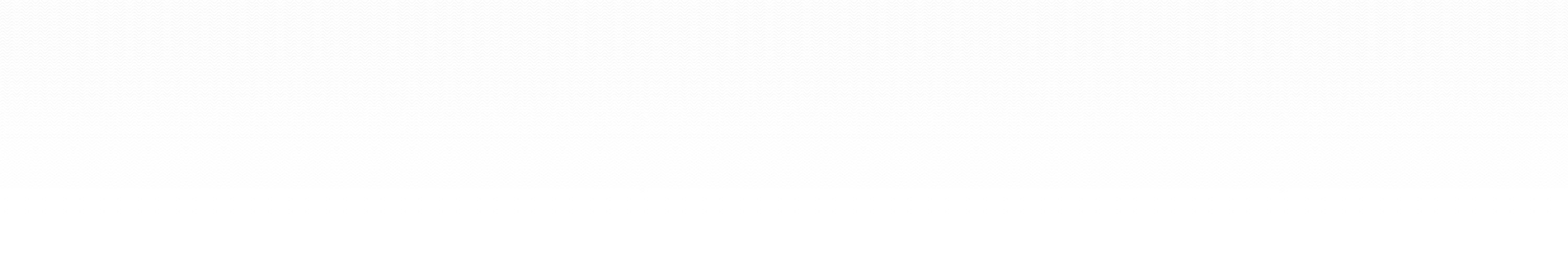### Fall 2013

MATH 1003: Introduction to calculus I (course coordinator | see Desire2Learn)

### Winter 2013

MATH 1063: Enriched introduction to calculus II
MATH 3543: Differential geometry for geodesy students

### Fall 2012

MATH 1003: Introduction to calculus I (course coordinator)
MATH 6992: Quantum field theory (reading course with Jack Gegenberg)

### Winter 2012

MATH 3503: Differential equations for engineers (course coordinator)
MATH 4503/6503: Numerical solution of differential equations

### Winter 2011

MATH 3503: Differential equations for engineers (course coordinator)
MATH 4483: General relativity (reading course with Viqar Husain)
MATH 4503: Numerical solution of differential equations

### Fall 2010

MATH 2513: Multivariable calculus for engineers

### Winter 2010

MATH 3503: Differential equations for engineers
MATH 4503: Numerical solution of differential equations